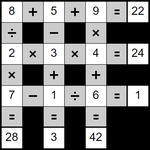# Daily MathemaGrids

Can you make the sums correct? Use all of the numbers 1 to 9 to complete the grid - the sums are solved strictly left to right, top to bottom.

## Today's Puzzles

Puzzle A 0 1 2 3 random hints
Puzzle B 0 1 2 3 random hints
Puzzle C 0 1 2 3 random hints

All daily items change at midnight GMT - set your local time zone.

## Objective / Rules• Complete the grid using all of the numbers 1 to 9.
• When completed all of the sums must be correct.
• The sums are solved strictly left to right, top to bottom.
• The normal order of mathematical operations is ignored.
• For example 2 + 5 x 9 is calculated as (2 + 5) x 9 = 63.
• ÷ 1 doesn't appear in the puzzle.
• x 1 doesn't appear in the puzzle (although there might be 1 x).
• At no point will any calculation go below zero, or become fractional.

Read the help / walkthrough page on MathemaGrids puzzles for the rules, a more detailed explanation, and a walkthrough.

Note: BrainBashers has a Dark Mode setting.